### Learning Outcomes

• Recognize characteristics of parabolas.
• Understand how the graph of a parabola is related to its quadratic function.

Curved antennas, such as the ones shown in the photo, are commonly used to focus microwaves and radio waves to transmit television and telephone signals, as well as satellite and spacecraft communication. The cross-section of the antenna is in the shape of a parabola, which can be described by a quadratic function.An array of satellite dishes. (credit: Matthew Colvin de Valle, Flickr)

## Characteristics of Parabolas

The graph of a quadratic function is a U-shaped curve called a parabola. One important feature of the graph is that it has an extreme point, called the vertex. If the parabola opens up, the vertex represents the lowest point on the graph, or the minimum value of the quadratic function. If the parabola opens down, the vertex represents the highest point on the graph, or the maximum value. In either case, the vertex is a turning point on the graph. The graph is also symmetric with a vertical line drawn through the vertex, called the axis of symmetry.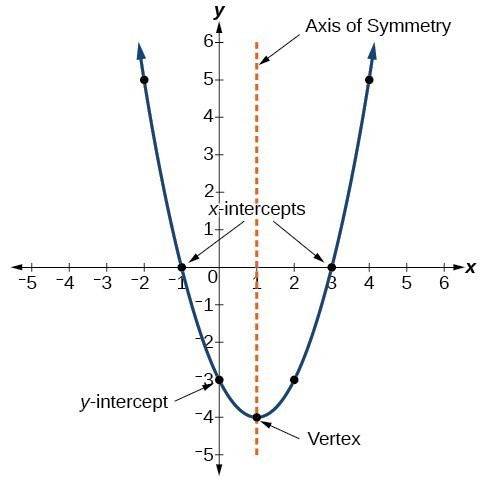The $y$-intercept is the point at which the parabola crosses the $y$-axis. The $x$-intercepts are the points at which the parabola crosses the $x$-axis. If they exist, the $x$-intercepts represent the zeros, or roots, of the quadratic function, the values of $x$ at which $y=0$.

### Example: Identifying the Characteristics of a Parabola

Determine the vertex, axis of symmetry, zeros, and y-intercept of the parabola shown below.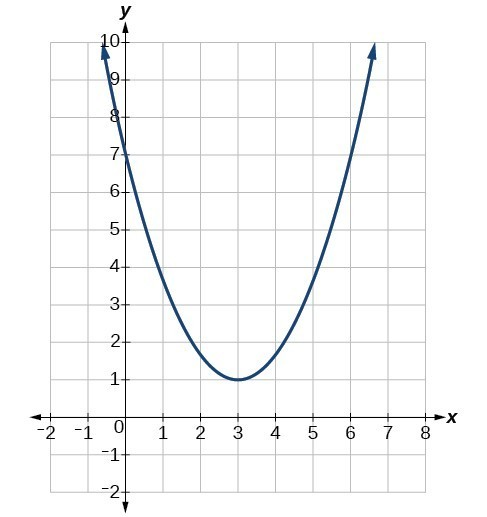The general form of a quadratic function presents the function in the form

$f\left(x\right)=a{x}^{2}+bx+c$

where $a$, $b$, and $c$ are real numbers and $a\ne 0$. If $a>0$, the parabola opens upward. If $a<0$, the parabola opens downward. We can use the general form of a parabola to find the equation for the axis of symmetry.

The axis of symmetry is defined by $x=-\dfrac{b}{2a}$. If we use the quadratic formula, $x=\dfrac{-b\pm \sqrt{{b}^{2}-4ac}}{2a}$, to solve $a{x}^{2}+bx+c=0$ for the $x$-intercepts, or zeros, we find the value of $x$ halfway between them is always $x=-\dfrac{b}{2a}$, the equation for the axis of symmetry.

The figure below shows the graph of the quadratic function written in general form as $y={x}^{2}+4x+3$. In this form, $a=1,\text{ }b=4$, and $c=3$. Because $a>0$, the parabola opens upward. The axis of symmetry is $x=-\dfrac{4}{2\left(1\right)}=-2$. This also makes sense because we can see from the graph that the vertical line $x=-2$ divides the graph in half. The vertex always occurs along the axis of symmetry. For a parabola that opens upward, the vertex occurs at the lowest point on the graph, in this instance, $\left(-2,-1\right)$. The $x$-intercepts, those points where the parabola crosses the $x$-axis, occur at $\left(-3,0\right)$ and $\left(-1,0\right)$.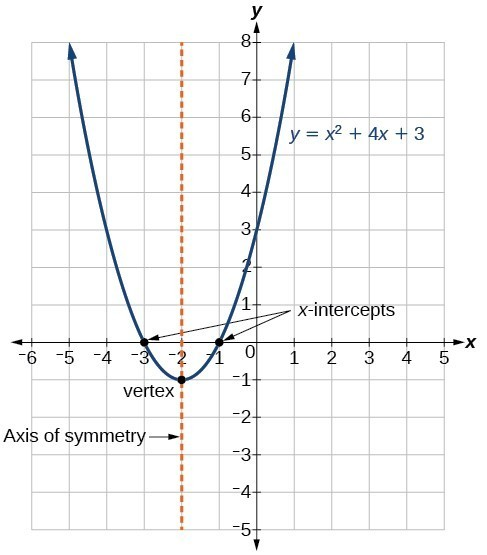The standard form of a quadratic function presents the function in the form

$f\left(x\right)=a{\left(x-h\right)}^{2}+k$

where $\left(h,\text{ }k\right)$ is the vertex. Because the vertex appears in the standard form of the quadratic function, this form is also known as the vertex form of a quadratic function.

## Given a quadratic function in general form, find the vertex of the parabola.

One reason we may want to identify the vertex of the parabola is that this point will inform us where the maximum or minimum value of the output occurs, $k$, and where it occurs, $h$. If we are given the general form of a quadratic function:

$f(x)=ax^2+bx+c$

We can define the vertex, $(h,k)$, by doing the following:

• Identify $a$, $b$, and $c$.
• Find $h$, the $x$-coordinate of the vertex, by substituting $a$ and $b$ into $h=-\dfrac{b}{2a}$.
• Find $k$, the $y$-coordinate of the vertex, by evaluating $k=f\left(h\right)=f\left(-\dfrac{b}{2a}\right)$

### Example: Finding the Vertex of a Quadratic Function

Find the vertex of the quadratic function $f\left(x\right)=2{x}^{2}-6x+7$. Rewrite the quadratic in standard form (vertex form).

### Try It

Given the equation $g\left(x\right)=13+{x}^{2}-6x$, write the equation in general form and then in standard form.

## Finding the Domain and Range of a Quadratic Function

Any number can be the input value of a quadratic function. Therefore the domain of any quadratic function is all real numbers. Because parabolas have a maximum or a minimum at the vertex, the range is restricted. Since the vertex of a parabola will be either a maximum or a minimum, the range will consist of all $y$-values greater than or equal to the $y$-coordinate of the vertex or less than or equal to the $y$-coordinate at the turning point, depending on whether the parabola opens up or down.

### A General Note: Domain and Range of a Quadratic Function

The domain of any quadratic function is all real numbers.

The range of a quadratic function written in general form $f\left(x\right)=a{x}^{2}+bx+c$ with a positive $a$ value is $f\left(x\right)\ge f\left(-\frac{b}{2a}\right)$, or $\left[f\left(-\frac{b}{2a}\right),\infty \right)$; the range of a quadratic function written in general form with a negative $a$ value is $f\left(x\right)\le f\left(-\frac{b}{2a}\right)$, or $\left(-\infty ,f\left(-\frac{b}{2a}\right)\right]$.

The range of a quadratic function written in standard form $f\left(x\right)=a{\left(x-h\right)}^{2}+k$ with a positive $a$ value is $f\left(x\right)\ge k$; the range of a quadratic function written in standard form with a negative $a$ value is $f\left(x\right)\le k$.

### How To: Given a quadratic function, find the domain and range.

1. The domain of any quadratic function as all real numbers.
2. Determine whether $a$ is positive or negative. If $a$ is positive, the parabola has a minimum. If $a$ is negative, the parabola has a maximum.
3. Determine the maximum or minimum value of the parabola, $k$.
4. If the parabola has a minimum, the range is given by $f\left(x\right)\ge k$, or $\left[k,\infty \right)$. If the parabola has a maximum, the range is given by $f\left(x\right)\le k$, or $\left(-\infty ,k\right]$.

### Example: Finding the Domain and Range of a Quadratic Function

Find the domain and range of $f\left(x\right)=-5{x}^{2}+9x - 1$.

### Try It

Find the domain and range of $f\left(x\right)=2{\left(x-\dfrac{4}{7}\right)}^{2}+\dfrac{8}{11}$.

The standard form of a quadratic function presents the function in the form

$f\left(x\right)=a{\left(x-h\right)}^{2}+k$

where $\left(h,k\right)$ is the vertex. Because the vertex appears in the standard form of the quadratic function, this form is also known as the vertex form of a quadratic function.

The standard form is useful for determining how the graph is transformed from the graph of $y={x}^{2}$. The figure below is the graph of this basic function.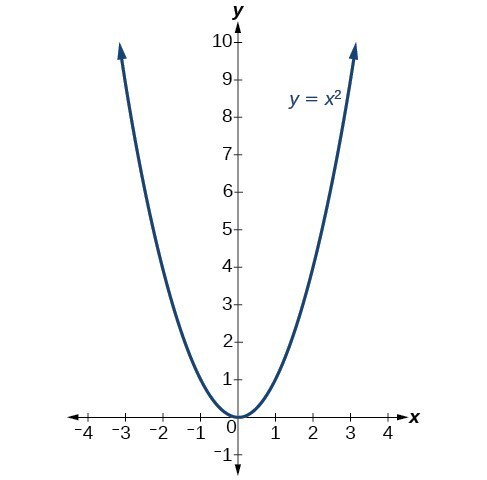## Shift Up and Down by Changing the Value of $k$

You can represent a vertical (up, down) shift of the graph of $f(x)=x^2$ by adding or subtracting a constant, $k$.

$f(x)=x^2 + k$

If $k>0$, the graph shifts upward, whereas if $k<0$, the graph shifts downward.

### Example

Determine the equation for the graph of $f(x)=x^2$ that has been shifted up 4 units. Also, determine the equation for the graph of $f(x)=x^2$ that has been shifted down 4 units.

## Shift left and right by changing the value of $h$

You can represent a horizontal (left, right) shift of the graph of $f(x)=x^2$ by adding or subtracting a constant, $h$, to the variable $x$, before squaring.

$f(x)=(x-h)^2$

If $h>0$, the graph shifts toward the right and if $h<0$, the graph shifts to the left.

### Example

Determine the equation for the graph of $f(x)=x^2$ that has been shifted right 2 units. Also, determine the equation for the graph of $f(x)=x^2$ that has been shifted left 2 units.

## Stretch or compress by changing the value of $a$.

You can represent a stretch or compression (narrowing, widening) of the graph of $f(x)=x^2$ by multiplying the squared variable by a constant, $a$.

$f(x)=ax^2$

The magnitude of $a$ indicates the stretch of the graph. If $|a|>1$, the point associated with a particular $x$-value shifts farther from the $x$axis, so the graph appears to become narrower, and there is a vertical stretch. But if $|a|<1$, the point associated with a particular $x$-value shifts closer to the $x$axis, so the graph appears to become wider, but in fact there is a vertical compression.

### Example

Determine the equation for the graph of $f(x)=x^2$ that has been compressed vertically by a factor of $\frac{1}{2}$. Also, determine the equation for the graph of $f(x)=x^2$ that has been vertically stretched by a factor of 3.

The standard form and the general form are equivalent methods of describing the same function. We can see this by expanding out the general form and setting it equal to the standard form.

\begin{align}&a{\left(x-h\right)}^{2}+k=a{x}^{2}+bx+c\\ &a{x}^{2}-2ahx+\left(a{h}^{2}+k\right)=a{x}^{2}+bx+c \end{align}
For the two sides to be equal, the corresponding coefficients must be equal. In particular, the coefficients of $x$ must be equal.
$-2ah=b,\text{ so }h=-\dfrac{b}{2a}$.

This is the $x$ coordinate of the vertexr and $x=-\dfrac{b}{2a}$ is the axis of symmetry we defined earlier. Setting the constant terms equal gives us:

\begin{align}a{h}^{2}+k&=c \\[2mm] k&=c-a{h}^{2} \\ &=c-a-{\left(\dfrac{b}{2a}\right)}^{2} \\ &=c-\dfrac{{b}^{2}}{4a} \end{align}

In practice, though, it is usually easier to remember that $h$ is the output value of the function when the input is $h$, so $f\left(h\right)=f\left(-\dfrac{b}{2a}\right)=k$.

### Try It

A coordinate grid has been superimposed over the quadratic path of a basketball in the picture below. Find an equation for the path of the ball. Does the shooter make the basket?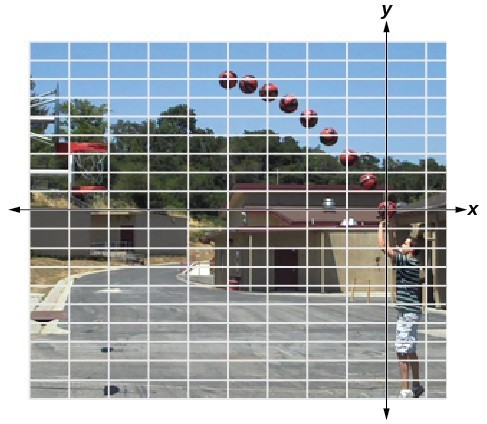(credit: modification of work by Dan Meyer)

## Key Concepts

• A polynomial function of degree two is called a quadratic function.
• The graph of a quadratic function is a parabola. A parabola is a U-shaped curve that can open either up or down.
• The axis of symmetry is the vertical line passing through the vertex.
• Quadratic functions are often written in general form. Standard or vertex form is useful to easily identify the vertex of a parabola. Either form can be written from a graph.
• The vertex can be found from an equation representing a quadratic function.
• The domain of a quadratic function is all real numbers. The range varies with the function.

## Glossary

axis of symmetry
a vertical line drawn through the vertex of a parabola around which the parabola is symmetric; it is defined by $x=-\dfrac{b}{2a}$.
general form of a quadratic function
the function that describes a parabola, written in the form $f\left(x\right)=a{x}^{2}+bx+c$, where $a$, $b$, and $c$ are real numbers and $a\ne 0$.
standard form of a quadratic function
the function that describes a parabola, written in the form $f\left(x\right)=a{\left(x-h\right)}^{2}+k$, where $\left(h,\text{ }k\right)$ is the vertex.